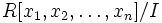# Affine ring

Let$R$ be a commutative unital ring. An affine ring over$R$ is a ring isomorphic to$R[x_1,x_2,\ldots,x_n]/I$ where$I$ is an ideal.
When we simply say affine ring, we may mean affine ring over a field, viz affine ring where the ring$R$ is a field.